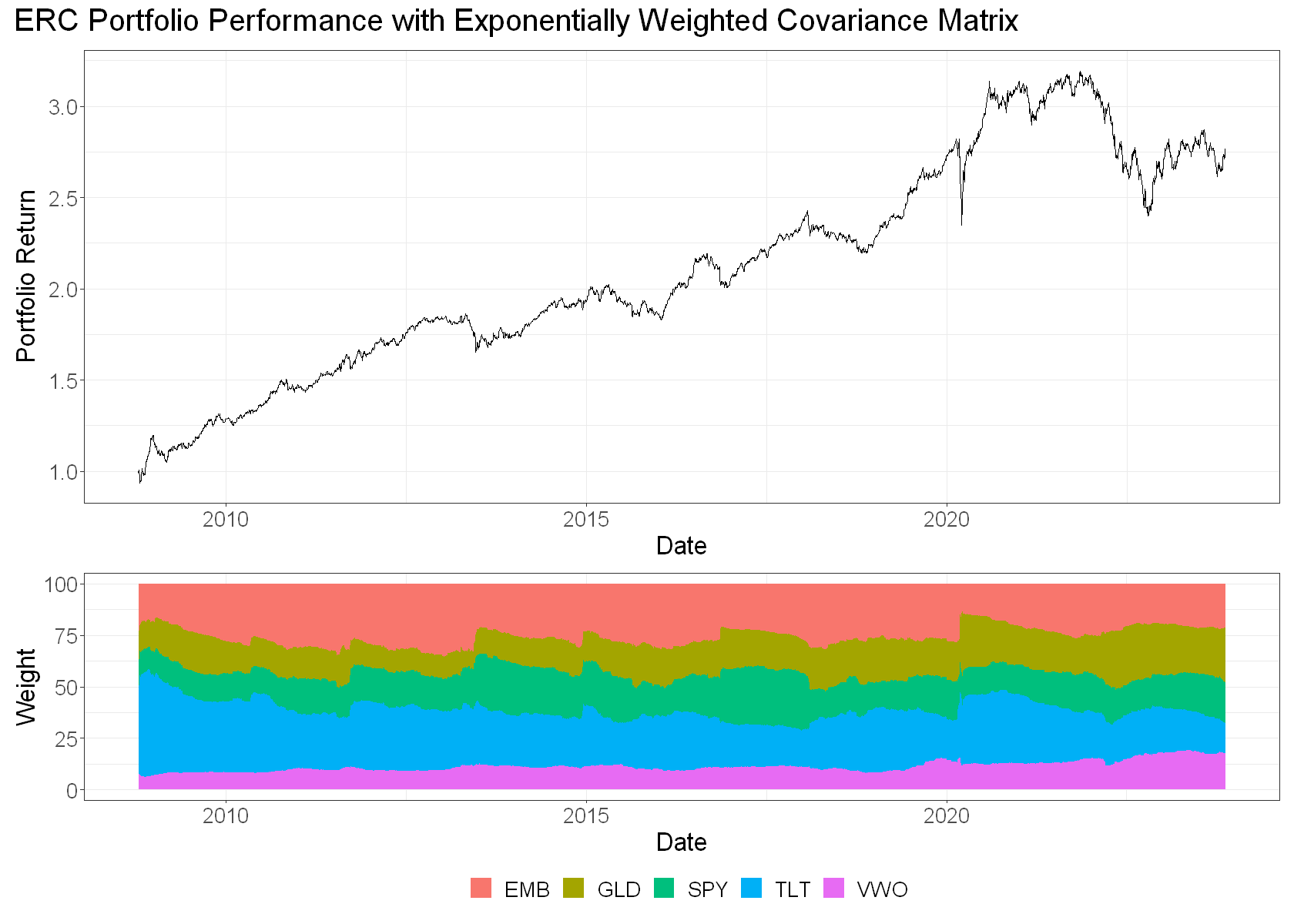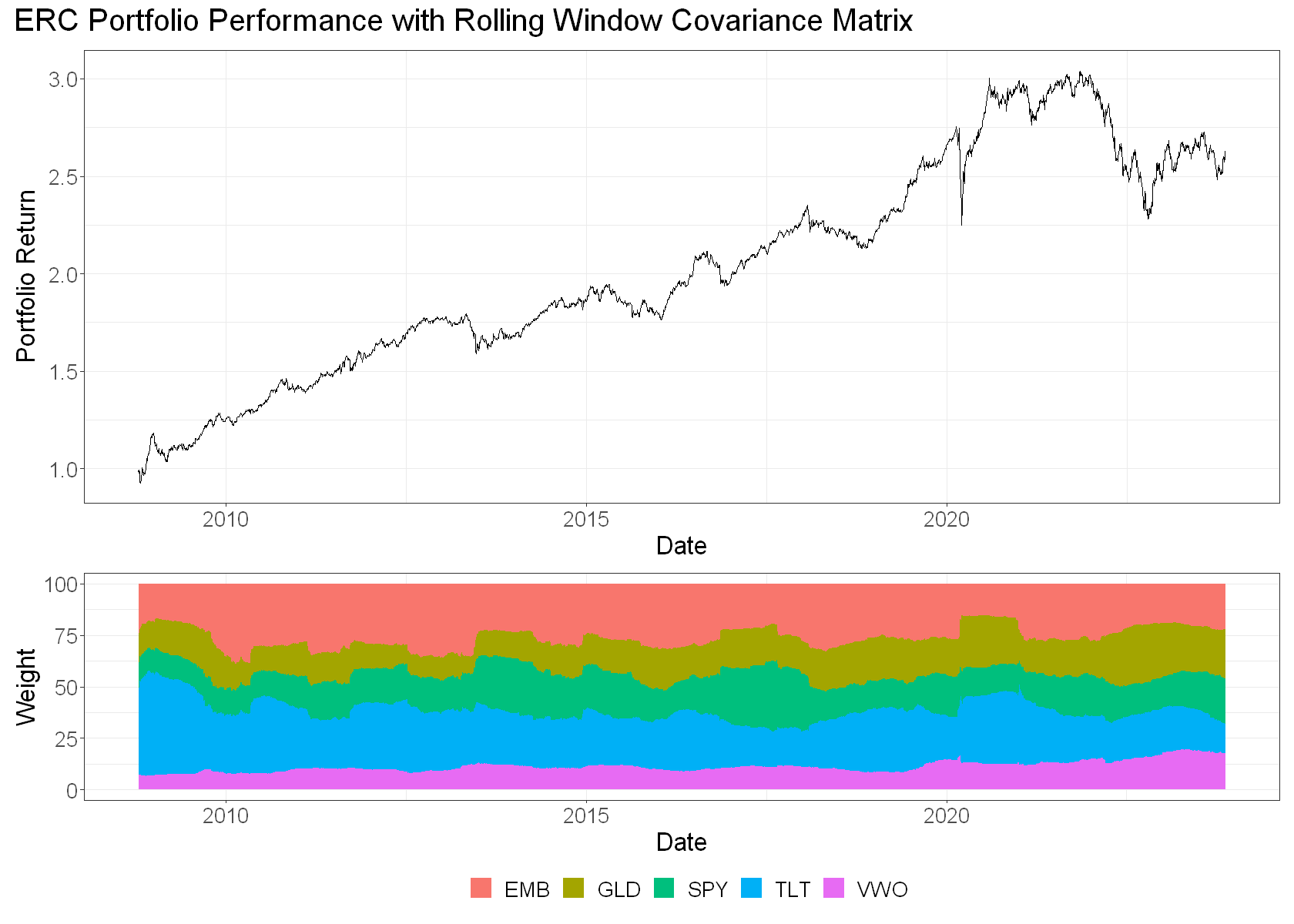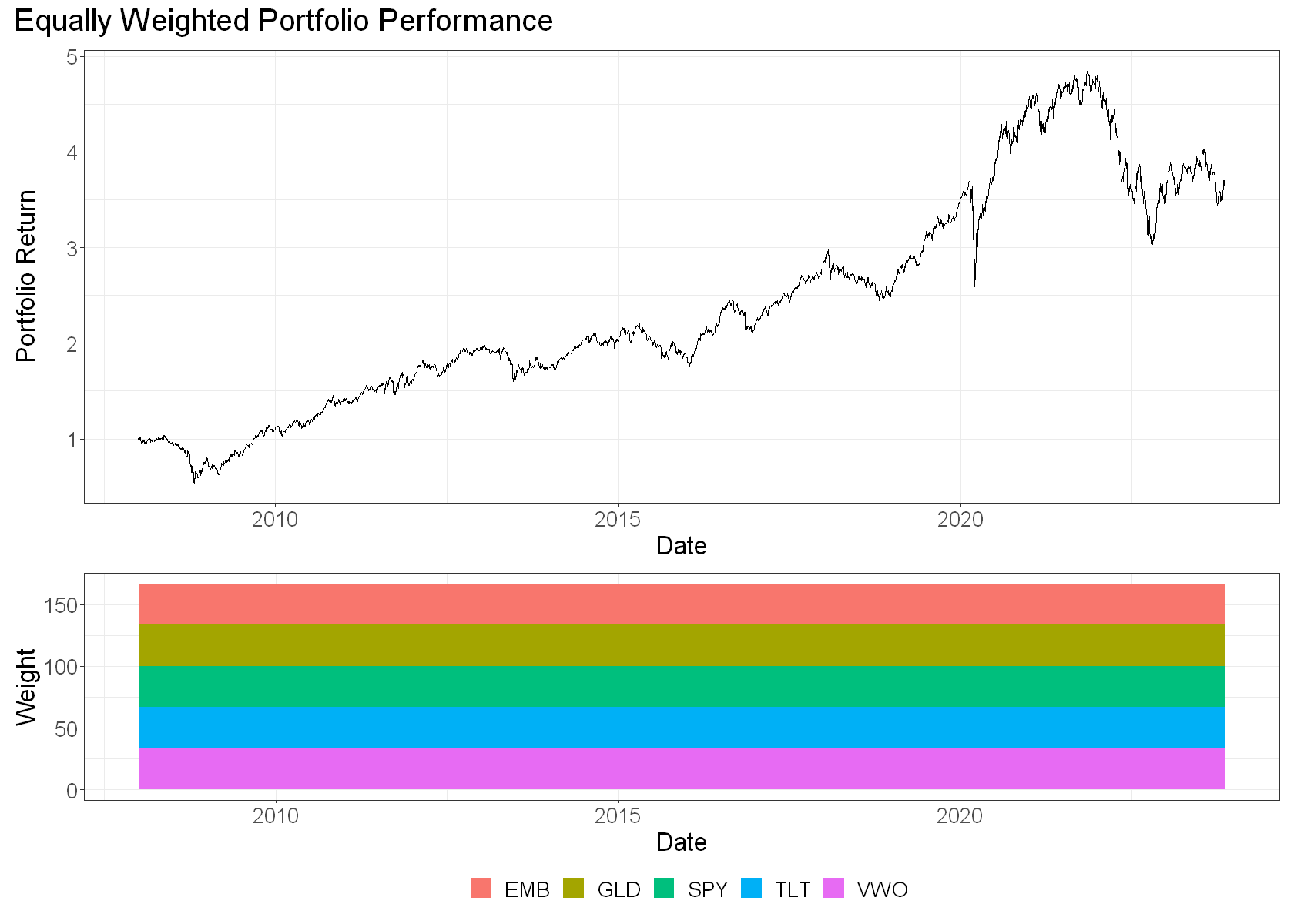# Exponentially weighted covariance in an Equal Risk Contribution portfolio optimisation problem

The Equal Risk Contribution (ERC) portfolio seeks to maximally diversify portfolio risk by equalising the risk contribution of each component.

The intuition is as follows:

• Imagine we have a 3-asset portfolio
• Assets 1 and 2 are perfectly correlated (correlation of 1.0)
• Asset 3 is uncorrelated with the other two (correlation of 0.0)

Let’s say we equal-weighted the three assets. We’d have 33% in asset 1, 33% in asset 2, and 33% in asset 3. But since assets 1 and 2 are perfectly correlated, from a risk perspective, this is like having 66% in a single asset and 33% in another (if we assume the variances are equal).

The ERC approach recognises that assets 1 and 2 are essentially two versions of the same thing, and would therefore down-weight these assets, and up-weight asset 3 until the risk contribution of each asset was equal. The actual weights would depend upon the variances of the individual assets, and their pairwise covariances.

Note that this optimisation doesn’t depend on expected returns – it only seeks to maximally diversify risk. This might make it a useful framework for strategies where you don’t have a view on which assets you expect to outperform, but where managing risk matters, for instance risk premia harvesting portfolios.

Here’s the original ERC paper if you’d like to read more.

The ERC optimisation depends on your estimate of covariance between the assets in the portfolio, and the optimisation is only as good as your estimate of future covariance.

We know from previous work that covariance is noisily autocorrelated. We also saw that an exponentially weighted measurement of past covariance may have some advantages over a rolling window estimate. It’s my working hypothesis that using an exponentially weighted covariance estimate should give ERC portfolios a small boost, since they do a marginally better job of estimating future covariance.

Let’s see if there’s anything to this idea.

We’ll create a daily-updating ERC portfolio using a reasonably diversified portfolio of liquid ETFs:

• TLT: long duration US government bonds
• SPY: US stocks
• GLD: gold
• VWO: emerging market stocks
• EMB: emerging market bonds

And we’ll see if an exponentially weighted covariance estimate leads to a better performing ERC portfolio.

An optimisation routine that figures out the ERC weights given a covariance matrix is implemented in the PERC function of R’s FRAPO (Financial Risk Modelling and Portfolio Optimisation) package. We’ll use it to calculate our ERC weights.

We’ll source some helper functions rather than include them in this notebook. I’ll include them below so that you can reproduce this if you want to.

library(FRAPO)
library(tidyverse)
library(roll)

# Set chart options
options(repr.plot.width = 14, repr.plot.height=7, warn=-1)
theme_set(theme_bw())
theme_update(text = element_text(size = 20))

# source helper functions
source("erc_helpers.R")

Get some data from Yahoo Finance and calculate returns.

# get data
tickers <- c("TLT", "SPY", "GLD", "VWO", "EMB")
prices <- yahoo_prices(
tickers,
from_date = "2008-01-01",
to_date = "2023-11-16"
) %>%
arrange(Date, Ticker)

head(prices)
A data.frame: 6 × 8
<date><chr><dbl><dbl><dbl><dbl><dbl><int>
12007-12-31EMB101.37101.40101.35101.35 47.42955 1600
22007-12-31GLD 82.74 82.83 81.98 82.46 82.46000 3815600
32007-12-31SPY147.10147.61146.06146.21107.71794108126800
42007-12-31TLT 92.78 93.28 92.60 93.04 59.36024 1647600
52007-12-31VWO 53.09 53.24 52.15 52.15 34.10643 1039000
62008-01-02EMB101.15101.53101.15101.49 47.49503 7600
# we'll need simple and log returns
returns <- prices %>%
arrange(Date) %>%
group_by(Ticker) %>%
mutate(
)

# make wide returns dataframe
wide_rets <- returns %>%
select(Ticker, Date, log_return) %>%
pivot_wider(Date, names_from = Ticker, values_from = log_return) %>%
na.omit()

# make pairwise returns dataframe
pairwise_returns <- returns %>%
full_join(returns, by = "Date") %>%
ungroup() %>%
# get all combinations (tickers) and remove duplicate combos (eg A-AA, AA-A)
mutate(tickers = ifelse(Ticker.x < Ticker.y, glue("{Ticker.x}, {Ticker.y}"), glue("{Ticker.y}, {Ticker.x}"))) %>%
distinct(Date, tickers, .keep_all = TRUE) %>%
# arrange for rolling pairwise ewma correlations
arrange(Date, .by_group = TRUE) %>%
na.omit()

head(pairwise_returns)
A tibble: 6 × 14
<chr><date><dbl><dbl><dbl><dbl><dbl><chr><dbl><dbl><dbl><dbl><dbl><chr>
EMB2008-01-0247.495030.0013805530.0017739750.0013796010.001772403EMB 47.49503 0.001380553 0.0017739750 0.001379601 0.0017724033EMB, EMB
EMB2008-01-0247.495030.0013805530.0017739750.0013796010.001772403GLD 84.86000 0.029105045 0.0083667098 0.028689536 0.0083319029EMB, GLD
EMB2008-01-0247.495030.0013805530.0017739750.0013796010.001772403SPY106.77493-0.008754419-0.0004828099-0.008792964-0.0004829265EMB, SPY
EMB2008-01-0247.495030.0013805530.0017739750.0013796010.001772403TLT 60.21521 0.014403125-0.0013782065 0.014300386-0.0013791571EMB, TLT
EMB2008-01-0247.495030.0013805530.0017739750.0013796010.001772403VWO 34.00834-0.002875910 0.0096153770-0.002880053 0.0095694435EMB, VWO
GLD2008-01-0284.860000.0291050450.0083667100.0286895360.008331903GLD 84.86000 0.029105045 0.0083667098 0.028689536 0.0083319029GLD, GLD

Next, we calculate exponentially weighted covariances for each pair of assets in the portfolio, for each day.

From that, we recover the covariance matrix each day, pass it to FRAPO::PERC and get the next day’s ERC weights.

This will take a while, as we calculate a new set of weights each day based on the updated covariance matrix:

# wrangle ewma covariances
lambda <- 0.99
wdw <- 200  # a reasonable approximation of an "equivalent" window length is 2/(1-lambda)
ewma_covs <- pairwise_returns %>%
group_by(tickers) %>%
arrange(Date, .by_group = TRUE) %>%
mutate(cov = ewma_cov(log_return.x, log_return.y, lambda = lambda, initialisation_wdw = wdw)) %>%
select(Date, tickers, cov) %>%
na.omit()

ewma_covs_long <- ewma_covs %>%
separate(tickers, into = c("ticker.x", "ticker.y"))

# daily ERC weights from exponentially weighted covariance matrix
days <- ewma_covs_long %>% distinct(Date) %>% pull()
tickers <- ewma_covs_long %>% distinct(ticker.x) %>% pull()
num_assets <- length(tickers)

erc_wgts <- vector(mode = "list", length = length(days))
non_psd <- vector(mode = "numeric", length = length(days))
i <- 1
for(day in days) {

cov_mat <- ewma_covs_long %>%
dplyr::filter(Date == day) %>%
recover_covmat(tickers, num_assets)

# check that covmat is PSD, if not convert to nearest
if(any(round(eigen(cov_mat)$values, 10) < 0)) { capture.output(psd <- Matrix::nearPD(cov_mat, keepDiag = TRUE, ensureSymmetry = TRUE, base.matrix = TRUE, maxit = 100), file = "NULL", type = "message") cov_mat <- psd$mat
# if still non-psd or non symmetrical (eg due to non-convergence of nearPD), use hacky method
if(any(round(eigen(cov_mat)$values, 10) < 0) || !isSymmetric(cov_mat)) { cov_mat <- get_near_psd(cov_mat) # sometimes this will still fail as we force symmetry - just note this for now if(any(round(eigen(cov_mat)$values, 10) < 0)) {
non_psd[[i]] <- day
}
}
}

# ERC weights based on covariance matrix
capture.output(these_wgts <- FRAPO::PERC(Sigma = cov_mat), file = "NULL")  # too much output...
erc_wgts[[i]] <- Weights(these_wgts)
i <- i + 1
}

erc_wgts <- wrangle_weights(erc_wgts, dates = ewma_covs_long %>% distinct(Date) %>% arrange(Date), tickers)

Let’s see how this approach performed (not considering costs):

options(repr.plot.width = 14, repr.plot.height=10)
show_performance(erc_wgts, title = "ERC Portfolio Performance with Exponentially Weighted Covariance Matrix")
A tibble: 1 × 3
Ann.ReturnAnn.VolAnn.Sharpe
<dbl><dbl><dbl>
0.071023240.085233560.8332779We get an average annual return of around 7.1% at an annualised volatility of 8.5% for a Sharpe of 0.83.

You can see in the weights plot how the ERC algorithm tilted our weights around as covariances evolved.

How does this compare to using a rolling covariance estimate?

# calculate rolling pairwise covariances over period as a long dataframe
wdw = 200 # a reasonable approximation of an "equivalent" window length is 2/(1-lambda)
rolling_covs <- pairwise_returns %>%
group_by(tickers) %>%
arrange(Date, .by_group = TRUE) %>%
mutate(cov = roll::roll_cov(  # replace with cov here, in previous function, and in previous use
x = log_return.x,
y = log_return.y,
width = wdw
)) %>%
select(Date, tickers, cov) %>%
na.omit()

rolling_covs_long <- rolling_covs %>%
separate(tickers, into = c("ticker.x", "ticker.y"))

# daily ERC weights from exponentially weighted covariance matrix
days <- rolling_covs_long %>% distinct(Date) %>% pull()
tickers <- rolling_covs_long %>% distinct(ticker.x) %>% pull()
num_assets <- length(tickers)

erc_wgts <- vector(mode = "list", length = length(days))
non_psd <- vector(mode = "numeric", length = length(days))
i <- 1
for(day in days) {

cov_mat <- rolling_covs_long %>%
dplyr::filter(Date == day) %>%
recover_covmat(tickers, num_assets)

# check that covmat is PSD, if not convert to nearest
if(any(round(eigen(cov_mat)$values, 10) < 0)) { capture.output(psd <- Matrix::nearPD(cov_mat, keepDiag = TRUE, ensureSymmetry = TRUE, base.matrix = TRUE, maxit = 100), file = "NULL", type = "message") cov_mat <- psd$mat
# if still non-psd or non symmetrical (eg due to non-convergence of nearPD), use hacky method
if(any(round(eigen(cov_mat)$values, 10) < 0) || !isSymmetric(cov_mat)) { cov_mat <- get_near_psd(cov_mat) # sometimes this will still fail as we force symmetry - just note this for now if(any(round(eigen(cov_mat)$values, 10) < 0)) {
non_psd[[i]] <- day
}
}
}

# ERC weights based on covariance matrix
capture.output(these_wgts <- FRAPO::PERC(Sigma = cov_mat), file = "NULL")  # too much output...
erc_wgts[[i]] <- Weights(these_wgts)
i <- i + 1
}

erc_wgts <- wrangle_weights(erc_wgts, dates = rolling_covs_long %>% distinct(Date) %>% arrange(Date), tickers)

show_performance(erc_wgts, title = "ERC Portfolio Performance with Rolling Window Covariance Matrix")
A tibble: 1 × 3
Ann.ReturnAnn.VolAnn.Sharpe
<dbl><dbl><dbl>
0.067772310.086244550.7858156We get an annual return of 6.7% at an annualised volatility of 8.6% for a Sharpe of 0.79. So our exponentially weighted covariance gave a small improvement for not much additional effort.

Compare to an equal weighted portfolio:

eq_wgts <- returns %>%
select(Date, Ticker, simple_return, fwd_simple_return) %>%
mutate(weight = 100/3)

show_performance(eq_wgts, title = "Equally Weighted Portfolio Performance")
A tibble: 1 × 3
Ann.ReturnAnn.VolAnn.Sharpe
<dbl><dbl><dbl>
0.10060950.1844160.5455575The equal weight version makes no attempt to equalise the risk of the portfolio’s component. It produces a slightly higher annual return (10%) at a much higher volatility (18%) for a much lower Sharpe (0.55).

It seems that you get the greatest improvement in risk adjusted returns simply from doing an ERC optimisation rather than equal weighting the portfolio components, and that a slightly better estimate of covariance gives a marginal improvement on that.

## Summary

• The Equal Risk Portfolio seeks to maximally diversify the portfolios based on an estimate of the components’ co-movements.
• This seems to significantly improve risk adjusted returns over the equal-weight case.
• Passing an exponentially weighted covariance estimate to the ERC optimiser seems to produce slightly better-performing weights than the rolling window covariance estimate.

### Further work

It would be interesting to look at the effect of larger and smaller values of lambda on the performance of the ERC portfolio, both before and after costs. Smaller values of lambda will lead to more hyperactive rebalancing. That will probably lead to better pre-cost performance, but worse after-cost performance. It would be interesting to explore this trade off between responsiveness and turnover.

Another example of using an exponentially weighted covariance estimate would be in a medium frequency convergence trade over a basket of related assets. In this example, you have a bunch of signals predicting expected returns, and you use a risk component to prevent getting too concentrated. Maybe we can do an example of this in a future post.

## Helper functions

To reproduce this notebook, place the following functions in a file named “erc_helpers.R” in the same directory as your notebook file. You’ll also need the functions for getting data from Yahoo Finance from this post. Note the path to these functions in the code below (line 7) and adjust as needed.

# helper functions for ew_covariance_erc_optimisation.ipynb

library(Matrix)
library(patchwork)

# functions for getting prices from Yahoo Finance
source("../data_tools/yahoo_prices.R")

# EWMA covariance estimate
# note definition of lambda in line with Risk Metrics
# ie higher values of lambda put less weight on the most recent returns and more weight on historical returns.
ewma_cov <- function(x, y, lambda, initialisation_wdw = 100) {
# TODO:
# check that x and y are the same length and greater than initialisation_wdw

# create initialisation window and estimation window
init_x = x[1:initialisation_wdw]
init_y = y[1:initialisation_wdw]

num_obs <- length(x)

# initial covariance and mean return estimates
old_cov <- cov(init_x, init_y)
old_x <- mean(init_x)
old_y <- mean(init_y)

# preallocate output vector
ewma_cov <- vector(mode = "numeric", length = num_obs)

# pad with NA for initialisation window
ewma_cov[1:initialisation_wdw] <- NA

# covariance estimate
for(i in c((initialisation_wdw+1):num_obs)) {
ewma_cov[i] <- lambda*old_cov + (1 - lambda)*(old_x * old_y)
old_cov <- ewma_cov[i]
old_x <- x[i]
old_y <- y[i]
}
ewma_cov
}

# function for recovering daily covmat from long covariance dataframe
#' @param long_covs: long dataframe with column cov for covariance
recover_covmat <- function(long_covs, tickers, num_assets) {
cov_mat <- matrix(rep(0, num_assets*num_assets), num_assets)
dimnames(cov_mat) <- list(tickers, tickers)

# recover lower triangle and diagonal
cov_mat[lower.tri(cov_mat, diag = TRUE)] <- long_covs$cov # recover upper triangle as upper triangle of transpose of half-formed matrix cov_mat[upper.tri(cov_mat)] <- t(cov_mat)[upper.tri(cov_mat)] cov_mat } # wrangle erc weights matrix into long dataframe with dates and returns wrangle_weights <- function(erc_wgts, dates, tickers) { erc_wgts <- as.data.frame(do.call(rbind, erc_wgts)) names(erc_wgts) <- tickers # add Date column and pivot long erc_wgts %>% bind_cols(dates) %>% pivot_longer(-Date, names_to = "Ticker", values_to = "weight") %>% # join returns left_join( returns %>% select(Ticker, Date, simple_return, fwd_simple_return), by = c("Ticker", "Date") ) } #' @param weights: long dataframe of Date, Ticker, weight, fwd_simple_return show_performance <- function(weights, title) { port_returns <- weights %>% na.omit() %>% group_by(Date) %>% summarise( port_return = sum(fwd_simple_return*weight/100.) ) portfolio_returns_plot <- port_returns %>% mutate(port_cum_return = cumprod(1+port_return)) %>% ggplot(aes(x = Date, y = port_cum_return)) + geom_line() + labs( x = "Date", y = "Portfolio Return" ) weights_plot <- weights %>% ggplot(aes(x = Date, y = weight, fill = Ticker)) + geom_area() + labs( x = "Date", y = "Weight", fill = "" ) + theme(legend.position = "bottom") plt <- portfolio_returns_plot / weights_plot + plot_annotation(title = title) + plot_layout(heights = c(2,1)) print(plt) port_returns %>% summarise( Ann.Return = 252*mean(port_return), Ann.Vol = sqrt(252)*sd(port_return), Ann.Sharpe = Ann.Return/Ann.Vol ) } #' @param weights: long dataframe of Date, Ticker, erc_wgt, fwd_simple_return calc_port_vol <- function(weights) { weights %>% na.omit() %>% group_by(Date) %>% summarise( port_return = sum(fwd_simple_return*weight/100.) ) %>% ungroup() %>% summarise(Ann.Vol = sqrt(252)*sd(port_return)) %>% pull(Ann.Vol) } # Function for making a PSD matrix. This is a bit hacky and we'll only use it when Matrix::nearPD fails to converge. get_near_psd <- function(mat) { # make symmetric mat = (mat + t(mat))/2 # for negative eigenvalues to zero eigs <- eigen(mat) eigs$values[eigs$values < 0] <- 0 # reconstruct PSD matrix from eigenvalues and eigenvectors near_psd <- eigs$vectors %*% diag(eigs$values) %*% t(eigs$vectors)
if(!isSymmetric(near_psd)) {
near_psd = (near_psd + t(near_psd))/2
}
near_psd
}